Download Presentation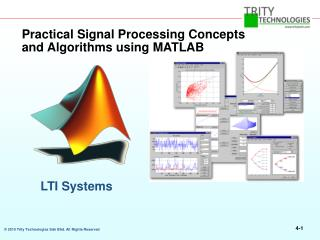Practical Signal Processing Concepts and Algorithms using MATLAB

# Practical Signal Processing Concepts and Algorithms using MATLAB - PowerPoint PPT Presentation

Practical Signal Processing Concepts and Algorithms using MATLAB. LTI Systems. Section Outline. LTI system representations The z-Transform Frequency and impulse response Introduction to filtering. Linear Time-Independent Systems. x(n). y(n). δ (n). h(n).I am the owner, or an agent authorized to act on behalf of the owner, of the copyrighted work described.
Download Presentation## Practical Signal Processing Concepts and Algorithms using MATLAB

An Image/Link below is provided (as is) to download presentation

Download Policy: Content on the Website is provided to you AS IS for your information and personal use and may not be sold / licensed / shared on other websites without getting consent from its author.While downloading, if for some reason you are not able to download a presentation, the publisher may have deleted the file from their server.

- - - - - - - - - - - - - - - - - - - - - - - - - - E N D - - - - - - - - - - - - - - - - - - - - - - - - - -
Presentation Transcript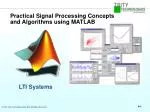LTI Systems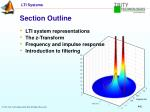Section Outline

• LTI system representations
• The z-Transform
• Frequency and impulse response
• Introduction to filtering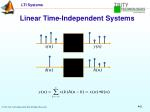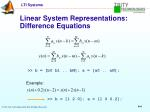Linear System Representations:Difference Equations

>> b = [b0 b1 .. bM]; a = [a0 .. aN];

Example:

>> b = [1 2 0]; a = [1 0 0.2];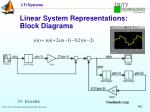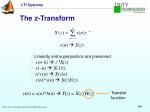The z-Transform

x(n)  X(z)

• Linearity and superposition are preserved
• x(n–k) z–kX(z)
• x(–n) X(1/z)
• anx(n) X(z/a)
• x(n)*y(n) X(z)Y(z)

y(n) = x(n)*h(n) Y(z) = X(z) H(z)

Transfer

function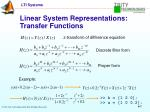Linear System Representations:Transfer Functions

z-transform of difference equation

Discrete filter form

Proper form

Example:

>> b = [1 2 0];

>> a = [1 0 0.2];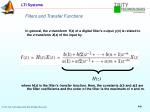Filters and Transfer Functions

In general, the z-transform Y(z) of a digital filter's output y(n) is related to the z-transform X(z) of the input by

where H(z) is the filter's transfer function. Here, the constants b(i) and a(i) are the filter coefficients and the order of the filter is the maximum of n and m.

H(z)For example let;

>>step = ones(50); %input data : step function

>>b = 1; % Numerator

>>a = [1 -0.9]; % Denominator

where the vectors b and a represent the coefficients of a filter in transfer function form. To apply this filter to your data, use

>> y = filter(b,a,step);

>> stem(y)

>>fvtool(b,a) %GUI.Don’t have to define input (if input is %step/impulse function)%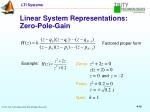Linear System Representations:Zero-Pole-Gain

Factored proper form

Zeros:z2 + 2z = 0

z(z + 2) = 0

z = 0andz = –2

Poles:z2 + 0.2 = 0

z = ± j

Gain:k = 1

Example:Zero-Pole-Gain Analysis

>> b = [1 2 0];

>> a = [1 0 0.2];

>> [z,p,k] = tf2zpk(b,a);

>> zplane(z,p)Zero-Pole Placement in Filter Design

x

o

passband

stopband

stable: inside

unit circle

minimum phase:

inside unit circle

x

o

x

x

o

no effect on

magnitude response

conjugate pairs

x

o

Steep transitions: stopband os paired with xs along same radial line

Causal system: number of os ≤ number of xsZero-Pole-Gain Editing in SPTool

• Choose Pole/Zero Editor design algorithm
• View system characteristics as you editImpulse Response

δ(n)

h(n)

>> impz(b,a,n,fs)Frequency Response

>> freqz(b,a,1,fs)Frequency Responseand the Transfer Function

Frequency response

Unwrapped response

x

o

xFilter Visualization Tool
• Magnitude response
• Phase response
• Group delay
• Impulse response
• Step response
• Pole-zero plot
• Filter coefficients

>> a = [1 0 .2];

>> b = [1 2 0];

>> fvtool(b,a)Filtering a Signal

>> y = filter(b,a,x)Filter Implementation and AnalysisConvolution and Filtering

The mathematical foundation of filtering is convolution. The MATLAB conv function performs standard one-dimensional convolution, convolving one vector with another

A digital filter's output y(k) is related to its input x(k) by convolution with its impulse response h(k).

>> x = [1 2 1];

>> h = [1 1 1];

>> y = conv(h,x);

>> stem(y)Importing a Filter into SPTool

Choose FileImport from the SPTool main menu.Applying a Filter in SPTool

Applying a Filter in SPTool

Select the signal and the filter

Choose the name of the output signal

Click on ApplyFilter Coefficients and Filter Names

In general, the z-transform Y(z) of a digital filter's output y(n) is related to the z-transform X(z) of the input by

• Many standard names for filters reflect the number of a and b coefficients present:
• When n=0 (that is, b is a scalar), the filter is an Infinite Impulse Response (IIR), all-pole, recursive, or autoregressive (AR) filter.
• When m=0 (that is, a is a scalar), the filter is a Finite Impulse Response (FIR), all-zero, nonrecursive, or moving-average (MA) filter.
• If both n and m are greater than zero, the filter is an IIR, pole-zero, recursive, or autoregressive moving-average (ARMA) filter.IIR Filter Design

• The primary advantage of IIR filters over FIR filters is that they typically meet a given set of specifications with a much lower filter order than a corresponding FIR filter.Section Summary

• LTI system representations
• The z-transform
• Frequency and impulse response
• Introduction to filtering